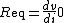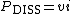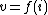# Negative-resistance circuits

Also found in: Dictionary.

## Negative-resistance circuits

Electronic circuits or devices that, over some range of voltage v and current i, satisfy Eq. (1)

(1)for equivalent resistance Req (where the voltage and current polarities are defined in Fig. 1a). They are used as building blocks in designing circuits for a wide range of applications, including amplifiers, oscillators, and memory elements. See Amplifier, Oscillator

An ideal negative resistor would have the voltage-current relationship (transfer characteristic) shown in Fig. 1b, and thus satisfy Ohm's law with a negative value for the resistance. However, the same effect can generally be obtained with any circuit (or physical device) whose voltage-current curve contains a region of negative slope. Figure 1c, for example, shows transfer characteristics typical of a tunnel diode and a neon bulb, which can be operated in the negative-resistance regions indicated. See Ohm's law

Common generalizations of the negative-resis­tance idea include negative capacitors, negative inductors, and frequency-dependent negative resistors. Some of the circuits used to implement them are negative impedance converters, negative impedance inverters, and generalized immittance converters. See Capacitor, Inductor

The power dissipated in a device, given by Eq. (2),

(2)is negative in the second and fourth quadrants of the v-i plane of Figs. 1b and c. Thus, the ideal negative resistor whose characteristic is shown in Fig. 1b generates power. Two consequences of this are that an active circuit (a circuit containing a power supply) is required to implement the ideal characteristic of Fig. 1b but is not necessary for the small-signal negative resistances of Fig. 1c; and that for any practical circuit, the characteristic curve must eventually fold over into the power-dissipating quadrants, as shown in Fig. 2a or b. If the curve did not fold but just continued forever, it would be possible to extract an infinite amount of power from the device.

The two types of curve of Fig. 2 correspond to an important dichotomy in types of negative resistance. The N-shaped curve of Fig. 2a allows current to be a single-valued function of voltage (but not vice versa), and circuits with this behavior are therefore called voltage-controlled negative resistors. Dually, the S-shaped curve of Fig. 2b, for which Eq. (3) is appropriate,

(3)describes a current-controlled negative resistor. The tunnel-diode characteristic of Fig. 1c can be seen to be voltage-controlled, while the neon tube is current-controlled.

If the terminals of a current-controlled negative resistor are open-circuited, then i = 0 and there is a unique solution v = f(0). The voltage-controlled circuit, however, can have any of three voltages in this situation (the three intersections of the N with the horizontal axis). Dually, the S-curve gives a device with multiple equilibrium states when short-circuited. When the dynamic behavior of these circuits is accounted for, it is found that some of these equilibria are stable and some are unstable. These stability considerations are essential to designing a negative-resistance circuit for a particular application.

Negative resistors can be implemented by using amplifiers in positive-feedback configurations. Figure 3a shows how a voltage amplifier with a gain of 2 can be used to simulate a grounded negative resistor, and Fig. 3b shows an operational-amplifier implementation of the same idea. See Operational amplifier

In the practical case of a clipping amplifier, which has the input-output characteristics shown in Fig. 3c, the resulting large-signal voltage-current behavior of the simulated resistor is as shown in the figure. This is a voltage-controlled resistor.

The best-known negative-resistance device is the tunnel diode. It is very useful because the phenomenon that it exploits is a quantum-mechanical effect that happens much more rapidly than most others in electronics.

A tunnel diode consists of two very heavily doped regions of a semiconducting material with a very abrupt junction between them. These regions, like any crystalline material, can contain electrons only with energies in certain bands. One side of the junction is doped to have a generous supply of electrons in a certain band of energies, while the other side has a great many vacancies (holes) for electrons in another band. As the applied voltage increases, the bands of electrons and holes on the two sides of the junction start to slide past one another, and eventually their region of overlap starts to decrease. Since quantum tunneling can occur only from an electron in the “supply” to a vacancy at the same energy, this reduction in overlap reduces the amount of charge flowing. Thus, an increasing voltage produces decreasing current, for a negative differential resistance like that shown in Fig. 1c. See Semiconductor rectifier

A number of other quantum electronic devices have been developed that also have negative-resistance characteristics. In particular, devices have been constructed that have two barriers (instead of the single barrier created by the tunnel-diode junction) and make use of resonant tunneling, where the spacing between the barriers creates a resonance for electrons at certain frequencies. This resonance, in turn, enhances the rate of tunneling. These devices are claimed to be useful at terahertz (1012 Hz) frequencies. See Semiconductor heterostructures, Tunnel diode

McGraw-Hill Concise Encyclopedia of Engineering. © 2002 by The McGraw-Hill Companies, Inc.
Site: Follow: Share:
Open / Close